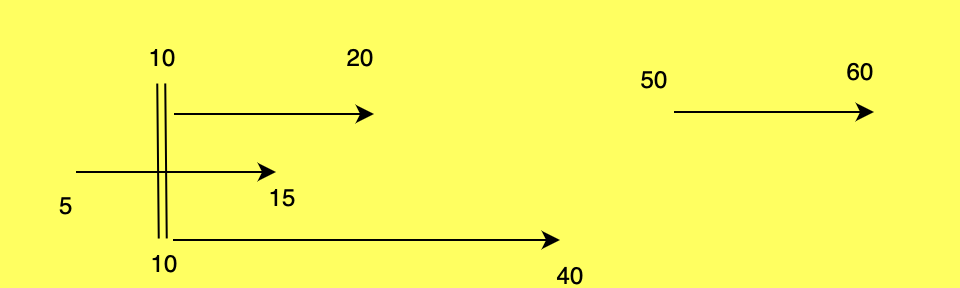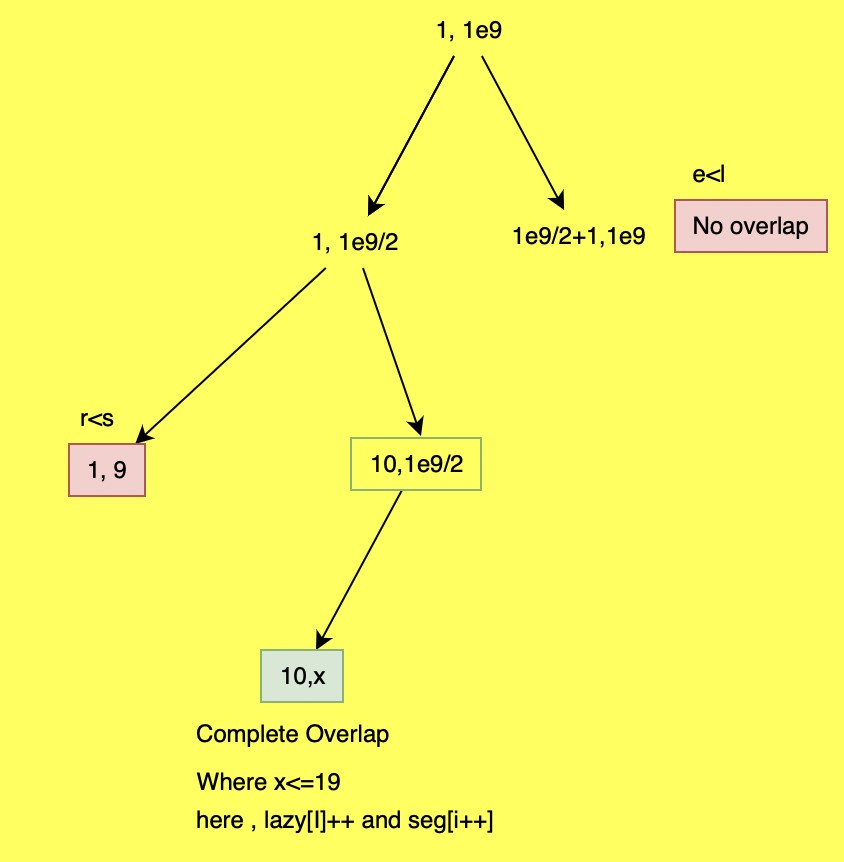×

Search anything:

# My Calendar III Problem [Solved with Segment Tree, Sweep Line]

#### Algorithms segment tree Data StructuresIn this article at OpenGenus, we have solved the Calendar 3 problem that is to find number of conflicting meetings in a given range of time. We have solved this using the concept of Sweep Line and Segment tree with Lazy Propagation.

1. Problem Description
2. Brute Force solution
3. Approach 1: Sweep-line Algorithm
4. Approach 2: Segment tree with Lazy propagation

Learn:

# Problem Description

You are given some events denoted as a set: [startTime, endTime]

After each event, return the maximum number of events/ meetings occuring between startTime and endTime-1 till the current query.

Implement the MyCalendarThree class:

• MyCalendarThree() is a constructor which Initializes the object.
• int book(int startTime, int endTime) Returns answer representing the maximum number of events occuring at a said time.

Example 1:

Input

[ [10, 20], [50, 60], [10, 40], [5, 15], [5, 10], [25, 55]]
Where each set is a query of (start time , end time)
Output
[ 1, 1, 2, 3, 3, 3]

Explanation

``````starting time = 10 , ending time = 20
myCalendarThree.book(10, 20); // return 1
starting time = 50 , ending time = 60
myCalendarThree.book(50, 60); // return 1
starting time = 10 , ending time = 40
myCalendarThree.book(10, 40); // return 2
starting time = 5 , ending time = 15
myCalendarThree.book(5, 15); // return 3
starting time = 5 , ending time = 10
myCalendarThree.book(5, 10); // return 3
starting time = 25 , ending time = 55
myCalendarThree.book(25, 55); // return 3
``````

Constraints:

0 <=startTime < endTime <= 1e9
At most 400 calls will be made to book.

In the problem at OpenGenus, we basically need to find maximum number of events occuring at a time. And here ending time is not inclusive , because the event ceases to run at end time.

# Brute Force solution

We basically have an array of all the times from 1 to 1e9, where we increment the events that are going on at that time for each query.Then we find maximum no of simultaneous events going on by traversing the array again.

This does work for 1e7 size but later the array cannot accomodate more memory thus gives runtime error. So we need to find a way that optimises space.

1. We have an array which stores all times from 0 to 100000000.
2. Every iteration the interval of [s,e) gets incremented by 1.
3. Then Max is calculated .
``````class MyCalendarThree {
public:
vector<int> v;
MyCalendarThree() {
v= vector<int>(1000000000);
}

int book(int s ,int e) {
for(int i=s ; i<e;i++){
v[i]++;
}
int ans=0;
for(int i:v)
ans = max(ans,i);
return ans;
}
};
#include<vector>
int main() {
int n = 6;
MyCalendarThree m;
vector<vector<int>> vi = {{10,20},{50,60},{10,40},{5,15},{5,10},{25,55}};
for(vector<int> i:vi){
int s=i,e=i;
// cin>>s>>e;
cout<<m.book(s,e)<<endl;

}
return 0;
}
``````

# Output:

``````Runtime Error

SIGKILL
``````
• Memory Limit is exceeded when size of vector is 1e9.
• Time Complexity - O(2*N^2)

# Approach 1: Sweep-line Algorithm

We saw in previous problem , when we were increasing the size of the vector to 1e9 the memory limit exceeded, so clearly vector is not feasible.Thus map can be used to do the same but updating across the complete range from starting time s to ending time e would take O(N) at worst case . We can just maintain the starting time and ending time and work accordingly , thus sweeping line algorithm does the same where we sweep an imaginary line (x or y axis) and solve the problem.Similar to minimum platforms problem , whenever at starting time s, no of events are incremented but at ending time e, as the event is finished it gets reduced.Thus this caters to imagination that there is a line from s to e-1. Then we calculate prefix sum on the updated map values and find out maximum simultaneous events occuring till date.

``````class MyCalendarThree {
public:
map<int,int> mp;//ordered map is necessary to get correct answer
MyCalendarThree() {

}

int book(int s, int e) {
mp[s]++; //at s time no of events increase.
mp[e]--;// at e time one event decreases as it ends.
int sum = 0,ans=0;
for(auto i : mp){
sum+=i.second;// calculate prefix sum i.e. no of overlapping events at a time.
ans = max(sum,ans);
}
return ans;
}
};
#include<vector>
int main() {
int n = 6;
MyCalendarThree m;
vector<vector<int>> v = {{10,20},{50,60},{10,40},{5,15},{5,10},{25,55}};
for(vector<int> i:v){
int s=i,e=i;
// cin>>s>>e;
cout<<m.book(s,e)<<endl;

}
return 0;
}

``````

# Result

``````1
1
2
3
3
3

``````

# Dry Run :

Eg: [10, 20], [50, 60], [10, 40], [5, 15], [5, 10], [25, 55]

1. for 10,20 - mp=1, mp=-1.When we traverse map , we get sum = sum+mp which is 1 and it remains maximum. Thus ans = 1
2. for 50,60 - mp=1,mp=-1,mp=1,mp=-1 again ans=1
3. for 10,40 - mp=2 so ans=2
4. for 5,15 - mp=1,mp=2 and so on , sum = mp+mp = 3 which would be the maximum
5. for 5,10 - mp=2,mp=1 so ans = 3
6. for 25,55 - ans will be 3 itself .

Time Complexity - O(N^2) where for each query it takes O(N) for traversing the for loop which can be at max 1e9 and for N Queries it takes O(N^2)

Space Complexity - O(N) size of map mp, which can be max 1e9.

# Approach 2: Segment tree with Lazy propagation

We can see that we are dealing with ranges between given two numbers , so in such cases segment tree can be useful as it can process between the ranges in logarithmic time and give answer. Segment tree is a tree in an array which stores corresponding answer for each range .

1. Lazy approach is used when we have multiple updates to do .
2. In lazy approach , certain updates are handled later . Only updates needed right now are made and rest and calculated later.
3. Lazy approach is useful for methods like finding min , max etc.
4. We maintain a segment tree map and lazy map,where segment tree will have number of events at particular time, lazy map will have all the number of events covering all times in the range.
5. Therefore segment tree will have size till max 1e9+7 as per the constraints given.

## Algorithm :

1. Create segment tree from 1 to 1e9.(as in constraints ending time can extend till 1e9)
2. Update the values accordingly in lazy , segment tree(seg) map respectively.
3. If the l,r are out of range which donot fall between or cover start time s and end time e will be rejected.
4. If completely within l and r then increment value of the current index .
5. If partially overlap then accordingly update l,mid and mid+1,r.
6. Then update current segment tree value with sum of max of left and right children and lazy array.
7. Return the seg which contains the overall result till now.
``````#include <iostream>
#include<unordered_map>
using namespace std;
class MyCalendarThree {
public:
unordered_map<int,int> seg;
unordered_map<int,int> lazy;
void update(int s,int e,int l,int r,int i){
if(s>r or e<l) return; //out of range
if(l>=s and r<=e){ // complete overlap
lazy[i]++;
seg[i]++;
}
else{//partial overlap
int m = (l+r)/2;
update(s,e,l,m,i*2);
update(s,e,m+1,r,i*2+1);
seg[i]=lazy[i]+max(seg[i*2],seg[i*2+1]);//update the values from the children and lazy values

}
}
int book(int startTime, int endTime) {
update( startTime,endTime-1,1,1000000000, 1);
return seg;
}
};
#include<vector>
int main() {
int n = 6;
MyCalendarThree m;
vector<vector<int>> v = {{10,20},{50,60},{10,40},{5,15},{5,10},{25,55}};
for(vector<int> i:v){
int s=i,e=i;
// cin>>s>>e;
cout<<m.book(s,e)<<endl;

}
return 0;
}

``````

# Result

``````
Output
1
1
2
3
3
3

``````

# Dry Run:

Eg: [10, 20], [50, 60], [10, 40], [5, 15], [5, 10], [25, 55]

1. for [10,20) : Since its not inclusive we take from 10-19the lazy array and seg array get updated for the cases from 10 to 19 , with rest all null as they are out of bounds.
Similarly , for the case [50,60).
2. for [10,40) , we already have lazy array which had been updated before from 10..19. Now seg[10..39] = max(seg[10..19],seg[20..39]) +lazy[10...39] which is 2 because the lazy values = 2 for 10...19 as it was 1 already before.
3. for [5,15) , we have lazy array updated as 2 from 10,14 , so now it will become 3.
Similar procedure for the rest of the queries or events.

Time Complexity - O(Nlogk) where k = 1e9, N no of queries and logk is the time complexity of update.
Space Complexity - O(Nlogk) where k = 1e9 max depth of segment tree os logk and for N queries

Thus we can see that this approach reduced complexity from N*N to N*logk which is very significant.

In this article at OpenGenus, we learnt about using lazy approach in segment tree and also sweeping algorithm and how to apply these concepts in Calendar problem.

#### Rashmitha

Final Year, B.tech (Information Technology ),Indira Gandhi Delhi Technical University For Women

My Calendar III Problem [Solved with Segment Tree, Sweep Line]